Next: Axisymmetric Case Up: Magnetohydrodynamics Previous: Flux Freezing   Contents

## Basic Equations of Ideal MHD

Basic equations are as follows: The mass continuity as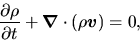(B.7)

the equation of motion as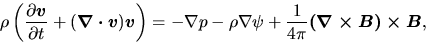(B.8)

the equation of thermal energy as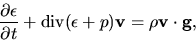(B.9)

or some barotropic relation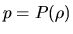and the induction equation as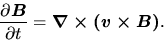(B.10)

Kohji Tomisaka 2007-07-08